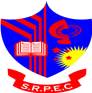Operating SystemDepartment of Computer Engineering

Operating System

Laboratory Incharge:
Prof. Megha B. Patel
Email: megha.comp@srpec.org

Experiments to be Performed:

An operating system (OS) is the software component of a computer system that is responsible for the management and coordination of activities and the sharing of the resources of the computer. We Perform the Practical related to scheduling Algo and Shell script Programming.

To understand the basic concepts of computer network and firm foundation for understanding how data communication occurring using computer network. It is based around the OSI Reference Model which deals with the major issues and related protocol studies in the various layers (Physical, Data Link, Network, Transport, Session, Presentation and Application) of the model. This course provides the student with fundamental knowledge of the various aspects of computer networking and enables students to appreciate recent developments in the area. The course will be driven from the engineering perspective.

• • Basic commands of UNIX.
• • Write a shell script to print a name of user.
• • Write a shell script to add two numbers.
• • Write a shell script to find a greater number out of two numbers.
• • Write a shell script to find a greater number out of three numbers.
• • Write a shell script to add, subtract, multiply and divide two numbers.
• • Write a shell script to generate marksheet of student. 1) Take three subjects. 2) Calculate percentage and a class obtained by the student.
• • Write a shell script to check the given number is even or odd.
• • Write a shell script to check whether the given number is prime or not.
• • Write a shell script to find Fibonacci series.
• • Write a shell script to find Factorial of given number.